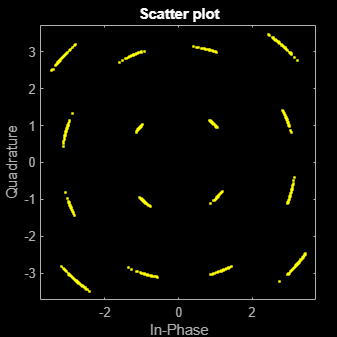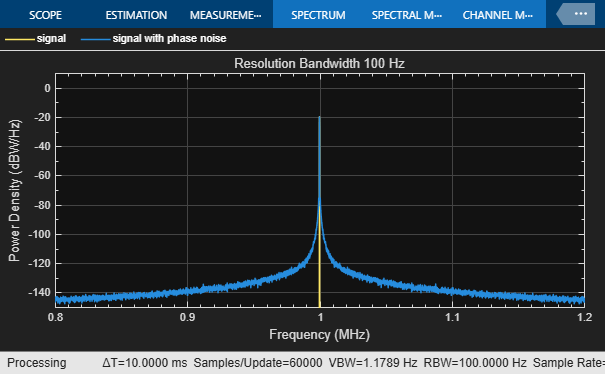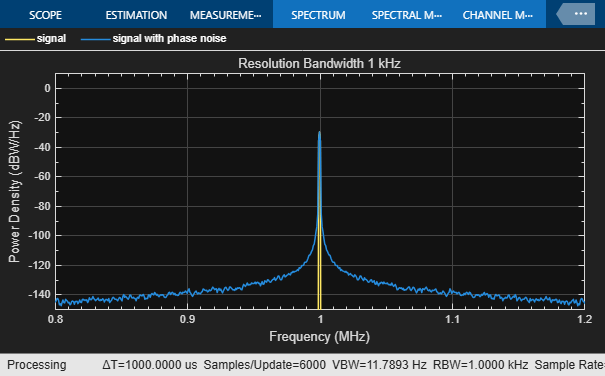# comm.PhaseNoise

Apply phase noise to baseband signal

## Description

The `comm.PhaseNoise` System object™ adds phase noise to a complex signal. This object emulates impairments introduced by the local oscillator of a wireless communication transmitter or receiver. The object generates filtered phase noise according to the specified spectral mask and adds it to the input signal. For a description of the phase noise modeling, see Algorithms.

To add phase noise using a `comm.PhaseNoise` object:

1. Create the `comm.PhaseNoise` object and set its properties.

2. Call the object with arguments, as if it were a function.

## Creation

### Syntax

``phznoise = comm.PhaseNoise``
``phznoise = comm.PhaseNoise(Name,Value)``
``phznoise = comm.PhaseNoise(level,offset,samplerate)``

### Description

````phznoise = comm.PhaseNoise` creates a phase noise System object with default property values.```

example

````phznoise = comm.PhaseNoise(Name,Value)` creates a phase noise object with the specified property `Name` set to the specified `Value`. You can specify additional name-value pair arguments in any order as (`Name1`,`Value1`,...,`NameN`,`ValueN`).```
````phznoise = comm.PhaseNoise(level,offset,samplerate)` creates a phase noise object with the phase noise level, frequency offset, and sample rate properties specified as value-only arguments. When specifying a value-only argument, you must specify all preceding value-only arguments.```

## Properties

expand all

Unless otherwise indicated, properties are nontunable, which means you cannot change their values after calling the object. Objects lock when you call them, and the `release` function unlocks them.

If a property is tunable, you can change its value at any time.

Phase noise level in decibels relative to carrier per hertz (dBc/Hz), specified as a vector of negative scalars. The `Level` and `FrequencyOffset` properties must have the same length.

Data Types: `double`

Frequency offset in Hz, specified as a vector of positive increasing values. The `Level` and `FrequencyOffset` properties must have the same length.

Data Types: `double`

Sample rate in samples per second, specified as a positive scalar. To avoid aliasing, the sample rate must be greater than twice the largest value specified by `FrequencyOffset`.

Data Types: `double`

Source of the random stream, specified as `'Global stream'` or `'mt19937ar with seed'`.If `RandomStream` is set to `'mt19937ar with seed'`, the mt19937ar algorithm is used for normally distributed random number generation, in which case the reset method reinitializes the random number stream to the value of the `Seed` property.

Data Types: `char` | `string`

Initial seed for `RandomStream`, specified as a positive scalar less than 232.

#### Dependencies

To enable this property, set `RandomStream` to `'mt19937ar with seed'`.

Data Types: `double`

## Usage

### Syntax

``out = phznoise(in)``

### Description

example

````out = phznoise(in)` adds phase noise, specified by the `phznoise` System object, to the input signal. The result is returned in `out`.```

### Input Arguments

expand all

Input signal, specified as an NS-by-1 vector of complex values. NS is the number of samples.

Data Types: `double`
Complex Number Support: Yes

### Output Arguments

expand all

Output signal, returned as an NS-by-1 vector of complex values. NS equals the number of samples in the input signal.

Data Types: `double`
Complex Number Support: Yes

## Object Functions

To use an object function, specify the System object as the first input argument. For example, to release system resources of a System object named `obj`, use this syntax:

`release(obj)`

expand all

 `visualize` Visualize spectrum mask of phase noise
 `step` Run System object algorithm `release` Release resources and allow changes to System object property values and input characteristics `reset` Reset internal states of System object

## Examples

collapse all

Add a phase noise vector and frequency offset vector to a 16-QAM signal. Then plot the signal.

Create a phase noise System object.

`pnoise = comm.PhaseNoise('Level',-50,'FrequencyOffset',20);`

Generate modulated symbols.

```M = 16; % From 16-QAM data = randi([0 M-1],1000,1); modData = qammod(data,M);```

Use `pnoise` to apply phase noise. Plot the impaired data.

```y = pnoise(modData); scatterplot(y)```View the effects of phase noise on a 10 MHz sine wave by using a spectrum analyzer. Adjust the resolution bandwidth of the spectrum analyzer to see its impact on the visualized spectral noise.

Initialize variables for the simulation.

```fc = 1e6; % Carrier frequency in Hz fs = 4e6; % Sample rate in Hz. phNzLevel = [-85 -118 -125 -145]; % Phase noise level in dBc/Hz phNzFreqOff = [1e3 9.5e3 19.5e3 195e3]; % Phase noise frequency offset in Hz Nspf = 6e6; % Number of Samples per frame freqSpan = 400e3; % Frequency span in Hz for spectrum computation```

Create sine wave, phase noise, and spectrum analyzer objects.

```sinewave = dsp.SineWave('Amplitude',1,'Frequency',fc,'SampleRate',fs, ... 'SamplesPerFrame',Nspf,'ComplexOutput',true); pnoise = comm.PhaseNoise('Level',phNzLevel, ... 'FrequencyOffset',phNzFreqOff,'SampleRate',fs); spectrumscopeRBW1 = dsp.SpectrumAnalyzer('NumInputPorts',2, ... 'SampleRate',fs,'FrequencySpan','Span and center frequency', ... 'CenterFrequency',fc,'Span',freqSpan,'RBWSource','Property', ... 'RBW',1,'SpectrumType','Power density','SpectralAverages',10, ... 'SpectrumUnits','dBW','YLimits',[-150 10], ... 'Title','Resolution Bandwidth 1 Hz','Position',[79 147 605 374]); spectrumscopeRBW10 = dsp.SpectrumAnalyzer('NumInputPorts',2, ... 'SampleRate',fs,'FrequencySpan','Span and center frequency', ... 'CenterFrequency',fc,'Span',freqSpan,'RBWSource','Property', ... 'RBW',10,'SpectrumType','Power density','SpectralAverages',10, ... 'SpectrumUnits','dBW','YLimits',[-150 10], ... 'Title','Resolution Bandwidth 10 Hz','Position',[685 146 605 376]);```

To analyze the spectrum and phase noise, the example includes two spectrum analyzer objects, with 1 Hz and 10 Hz resolution bandwidths, respectively. The spectrum analyzer objects use the default `Hann` windowing setting, the units are set to `dBW/Hz`, and the number of spectral averages is set to `10`.

```x = sinewave(); y = pnoise(x);```

When the resolution bandwidth is 1 Hz, the `dBW/Hz` view for the spectrum analyzer shows the tone at 0 dBW/Hz. The spectrum analyzer object corrects for the power spreading effect of the Hann windowing. Results show the visual average of the phase noise match the specified phase noise spectrum.

`spectrumscopeRBW1(x,y)`When the resolution bandwidth is 10 Hz, the `dBW/Hz` view for the spectrum analyzer shows the tone at -10 dBW/Hz. The tone energy of the sine wave is now spread across 10 Hz instead of 1 Hz, so the sine wave PSD level reduces by 10 dB. With the resolution bandwidth at 10 Hz, the visual average of the phase noise still achieves the phase noise defined by the phase noise object.

With the resolution bandwidth increased from 1 Hz to 10 Hz, the spectrum analyzer object still corrects for the power spreading effect of the Hann window, and it achieves better spectral averaging with the wider resolution bandwidth. For more information, see Why Use Windows?.

`spectrumscopeRBW10(x,y)`Calculate the RMS phase noise in degrees between the pure and noisy sine waves. In the general case, the pure signal must be time aligned with the noisy signal to accurately determine the phase error. However, in this case, the periodicity of the sine wave makes this step unnecessary.

```ph_err = unwrap(angle(y) - angle(x)); rms_ph_nz_deg = rms(ph_err)*180/pi(); sprintf('The computed RMS phase noise is %3.2f degrees.\n',rms_ph_nz_deg)```
```ans = 'The computed RMS phase noise is 0.18 degrees. ' ```

## Algorithms

The output signal, yk, is related to input sequence xk by yk=xkejφk, where φk is the phase noise. The phase noise is filtered Gaussian noise such that φk=f(nk), where nk is the noise sequence and f represents a filtering operation.To model the phase noise, define the power spectrum density (PSD) mask characteristic by specifying scalar or vector values for the frequency offset and phase noise level.

• For a scalar frequency offset and phase noise level specification, an IIR digital filter computes the spectrum mask. The spectrum mask has a 1/f characteristic that passes through the specified point.

• For a vector frequency offset and phase noise level specification, an FIR filter computes the spectrum mask. The spectrum mask is interpolated across log10(f). It is flat from DC to the lowest frequency offset, and from the highest frequency offset to half the sample rate.

IIR Digital Filter

For the IIR digital filter, the numerator coefficient is

`$\lambda =\sqrt{2\pi {f}_{offset}{10}^{L/10}}\text{\hspace{0.17em}},$`

where foffset is the frequency offset in Hz and L is the phase noise level in dBc/Hz. The denominator coefficients, γi, are recursively determined as

`${\gamma }_{i}=\left(i-2.5\right)\frac{{\gamma }_{i-1}}{i-1}\text{\hspace{0.17em}},$`

where γ1 = 1, i = {1, 2,..., Nt}, and Nt is the number of filter coefficients. Nt is a power of 2, from `2`7 to `2`19. The value of Nt grows as the phase noise offset decreases towards 0 Hz.

FIR Filter

For the FIR filter, the phase noise level is determined through log10(f) interpolation for frequency offsets over the range [df, fs / 2], where df is the frequency resolution and fs is the sample rate. The phase noise is flat from 0 Hz to the smallest frequency offset, and from the largest frequency offset to fs / 2. The frequency resolution is equal to $\frac{{f}_{s}}{2}\left(\frac{1}{{N}_{t}}\right)$, where Nt is the number of coefficients, and is a power of 2 less than or equal to `2`16. If Nt < `2`8, a time domain FIR filter is used. Otherwise, a frequency domain FIR filter is used.

The algorithm increases Nt until these conditions are met:

• The frequency resolution is less than the minimum value of the frequency offset vector.

• The frequency resolution is less than the minimum difference between two consecutive frequencies in the frequency offset vector.

• The maximum number of FIR filter taps is `2`16.

 Kasdin, N. J., "Discrete Simulation of Colored Noise and Stochastic Processes and 1/(f^alpha); Power Law Noise Generation." The Proceedings of the IEEE. Vol. 83, No. 5, May, 1995, pp 802–827.